Courses

# RD Sharma Solutions -Page No.9.18, Ratio Proportion And Unitary Method, Class 6, Maths Class 6 Notes | EduRev

## RD Sharma Solutions for Class 6 Mathematics

Created by: Abhishek Kapoor

## Class 6 : RD Sharma Solutions -Page No.9.18, Ratio Proportion And Unitary Method, Class 6, Maths Class 6 Notes | EduRev

The document RD Sharma Solutions -Page No.9.18, Ratio Proportion And Unitary Method, Class 6, Maths Class 6 Notes | EduRev is a part of the Class 6 Course RD Sharma Solutions for Class 6 Mathematics.
All you need of Class 6 at this link: Class 6

#### PAGE NO 9.18:

Question 1:  The price of 3 metres of cloth is Rs 79.50. Find the price of 15 metres of such cloth.

ANSWER: Price of 3 metres of cloth = Rs. 79.50

Price of 1 metre of cloth  = 79.50/3 = Rs.26.5

Price of 15 metres of cloth = 26.5×15 = Rs. 397.50

Question 2: The cost of 17 chairs is Rs 9605. Find the number of chairs that can be purchased in Rs 56500.

ANSWER: Number of chairs purchased for Rs. 9,605 = 17

Number of chairs purchased for Rs. 1 = 17/9605

Number of chairs purchased for Rs. 56,500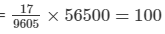Question 3: Three ferryloads are needed to carry 150 people across a river. How many people will be carried on 4 ferryloads?

ANSWER: Number of people needed to carry 3 ferryloads across a river =  150

Number of people needed to carry 1 ferryload across a river  = 150/3 = 50

Number of people needed to carry 4 ferryloads across a river =  50×4 = 200

Question 4: If 9 kg of rice costs Rs 120.60, What will 50 kg of such a quality of rice cost?

ANSWER: Cost of 9 kg rice = Rs. 120.60

Cost of 1 kg rice = 120.60/9 = Rs. 13.4

Cost of 50 kg rice = 13.4×50= Rs. 670

Question 5: A train runs 200 kilometres in 5 hours. How many kilometres does it run in 7 hours

ANSWER: Distance covered by the train in 5 hours = 200 km

Distance covered by the train in 1 hour  = 200/5 = 40 km

Distance covered by the train in 7 hours = 40×7 = 280

Question 6: 10 boys can dig a pitch in 12 hours. How long will 8 boys take to do it?

ANSWER: It is given that 10 boys can dig a pitch in 12 hours.

Therefore, time taken by one boy to dig the pitch = 10×12 = 120 hours

Thus, time taken by 8 boys to dig the same pitch  = 120/8 = 15 hours

Question 7: A man can work 8 hours daily and finishes a work in 12 days. If he works 6 hrs daily, in how many days will the same work be finished?

ANSWER: It is given that a man can work for 8 hours daily and finishes a work in 12 days.

Therefore, time taken by the man to finish the total work = 8×12=968×12=96 hours

If he works for 6 hours daily, the number of days required to finish the total work = 96/6 =16 days

Question 8: Fifteen post cards cost Rs 2.25. What will be the cost of 36 post cards? How many postcards can we buy in Rs 45?

ANSWER: Cost of 15 postcards = Rs. 2.25

Cost of 1 postcard = Rs. 2.25/15

Cost of 36 postcards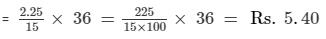Number of postcards purchased in Rs. 1 = 15/2.25

Number of postcards purchased in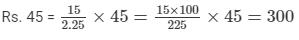Question 9: A rail journey of 75 km costs Rs 215. How much will a journey of 120 km cost?

ANSWER: Cost of a rail journey of 75 km = Rs. 215

Cost of a rail journey of 1 km  = Rs. 215/75

Cost of a rail journey of 120 km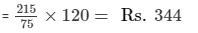Question 10: If the sales tax on a purchase worth Rs 60 is Rs 4.20. What wil be the sales tax on the purchase worth Rs 150?

ANSWER: Sales tax on a purchase worth Rs. 60 = Rs. 4.20

Sales tax on a purchase worth Rs. 1 = Rs. 420/60

Sales tax on a purchase worth Rs. 150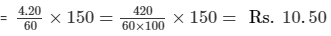Offer running on EduRev: Apply code STAYHOME200 to get INR 200 off on our premium plan EduRev Infinity!

103 docs

,

,

,

,

,

,

,

,

,

,

,

,

,

,

,

,

,

,

,

,

,

,

,

,

,

,

,

,

,

,

;